Anzeige

# RESTING MEMBRANE POTENTIAL.ppt

20. Mar 2023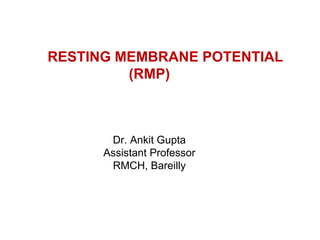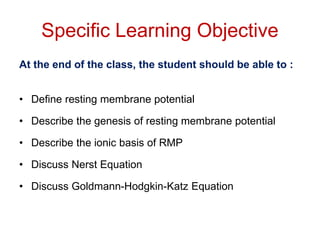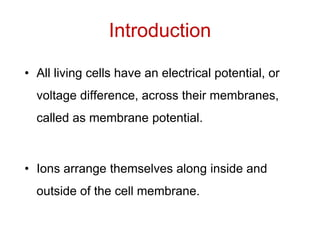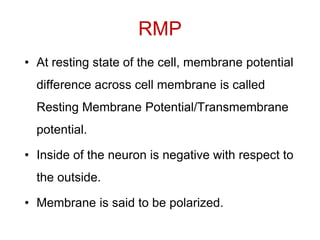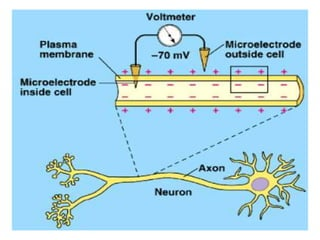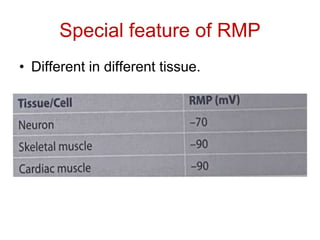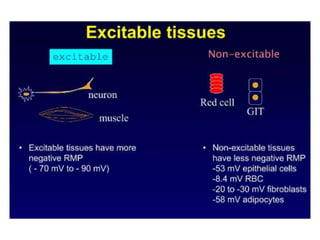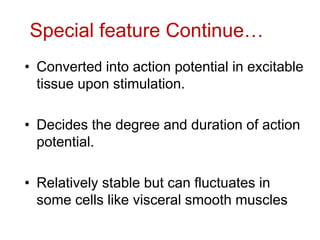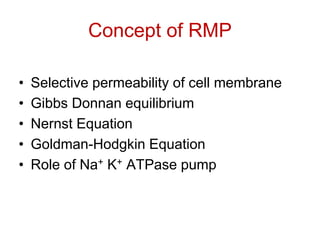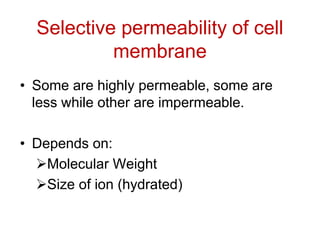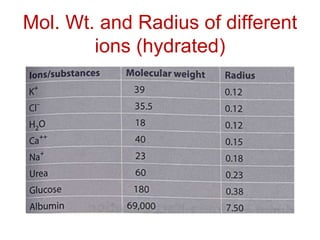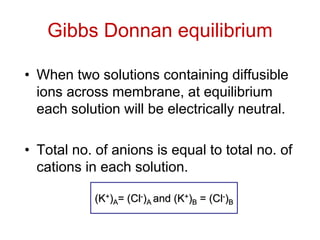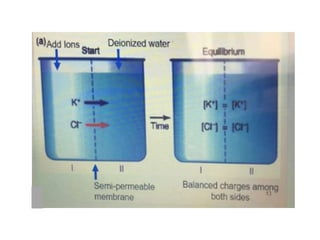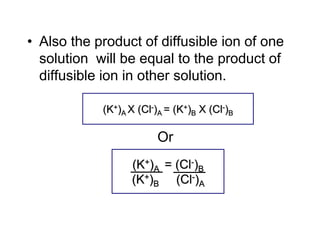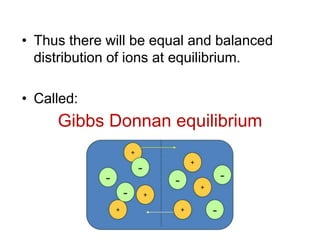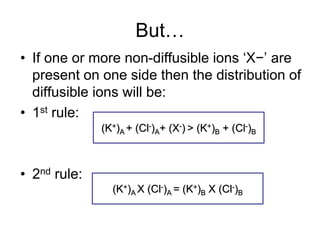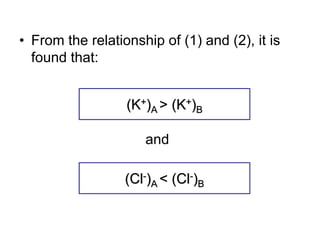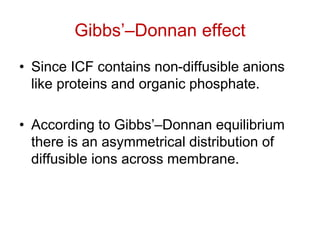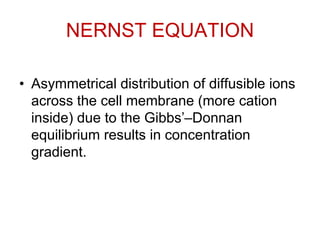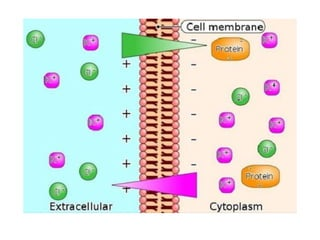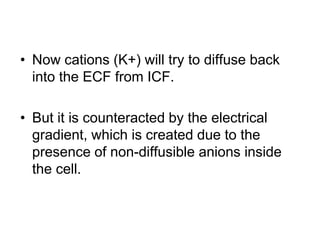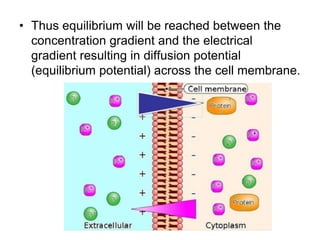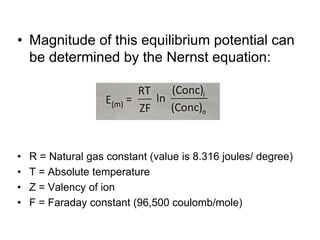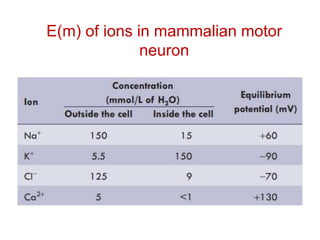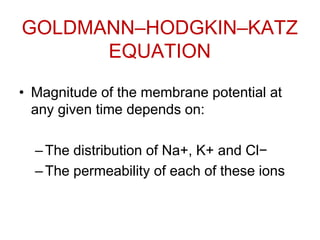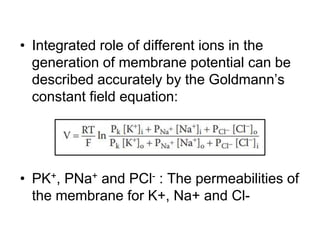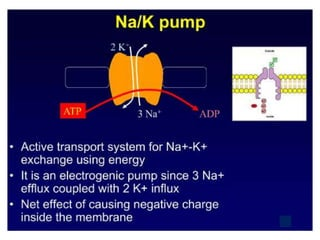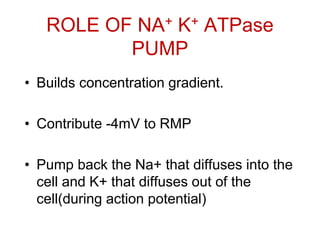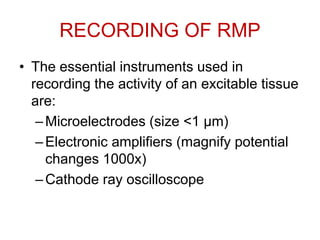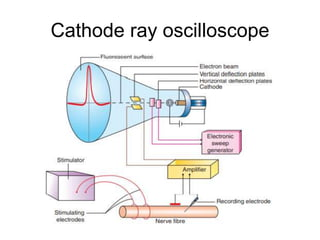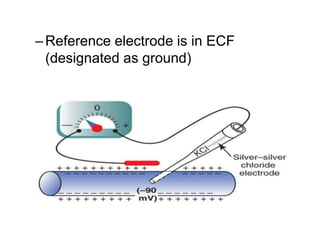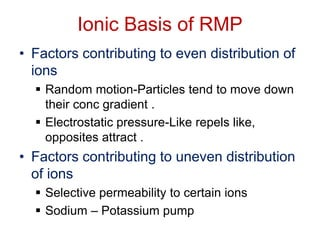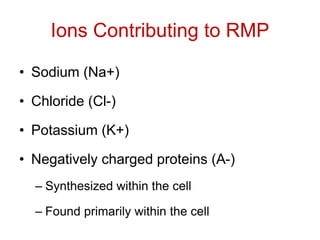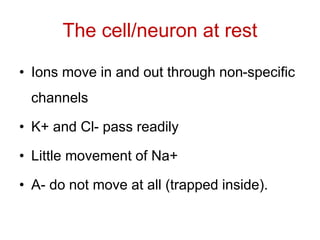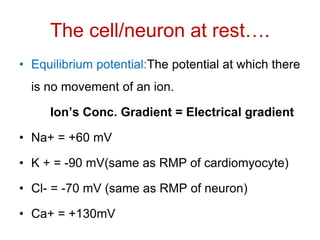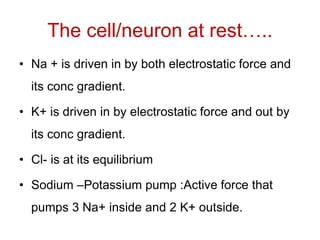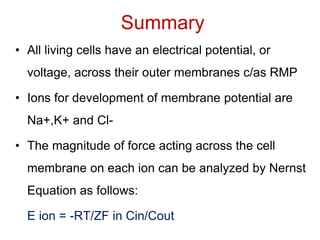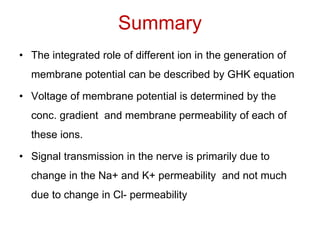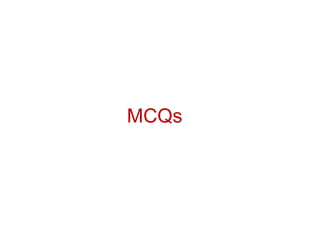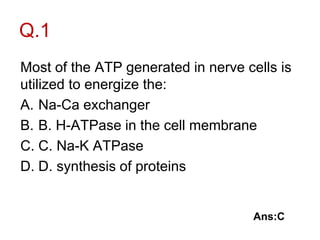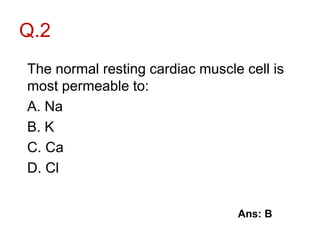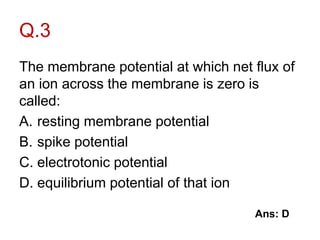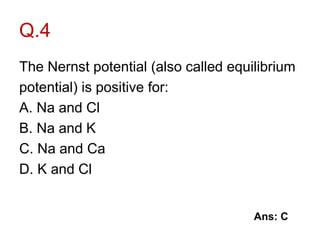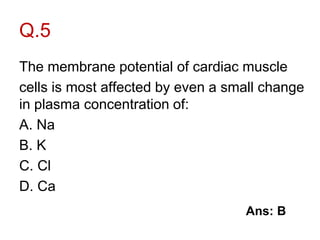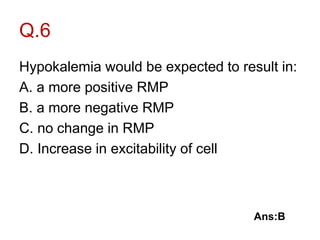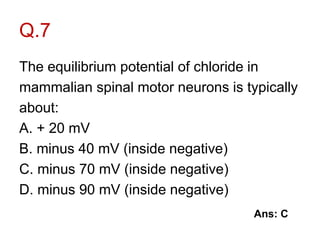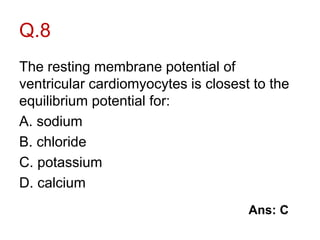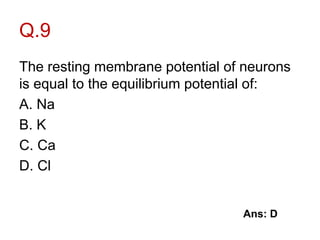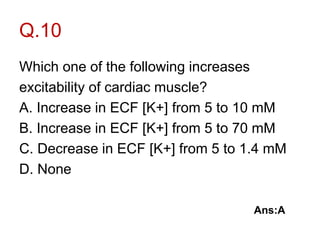1 von 51
Anzeige

### RESTING MEMBRANE POTENTIAL.ppt

1. RESTING MEMBRANE POTENTIAL (RMP) Dr. Ankit Gupta Assistant Professor RMCH, Bareilly
2. Specific Learning Objective At the end of the class, the student should be able to : • Define resting membrane potential • Describe the genesis of resting membrane potential • Describe the ionic basis of RMP • Discuss Nerst Equation • Discuss Goldmann-Hodgkin-Katz Equation
3. Introduction • All living cells have an electrical potential, or voltage difference, across their membranes, called as membrane potential. • Ions arrange themselves along inside and outside of the cell membrane.
4. RMP • At resting state of the cell, membrane potential difference across cell membrane is called Resting Membrane Potential/Transmembrane potential. • Inside of the neuron is negative with respect to the outside. • Membrane is said to be polarized.
5. Special feature of RMP • Different in different tissue.
6. Special feature Continue… • Converted into action potential in excitable tissue upon stimulation. • Decides the degree and duration of action potential. • Relatively stable but can fluctuates in some cells like visceral smooth muscles
7. Concept of RMP • Selective permeability of cell membrane • Gibbs Donnan equilibrium • Nernst Equation • Goldman-Hodgkin Equation • Role of Na+ K+ ATPase pump
8. Selective permeability of cell membrane • Some are highly permeable, some are less while other are impermeable. • Depends on: Molecular Weight Size of ion (hydrated)
9. Mol. Wt. and Radius of different ions (hydrated)
10. Gibbs Donnan equilibrium • When two solutions containing diffusible ions across membrane, at equilibrium each solution will be electrically neutral. • Total no. of anions is equal to total no. of cations in each solution. (K+)A= (Cl-)A and (K+)B = (Cl-)B
11. A B
12. • Also the product of diffusible ion of one solution will be equal to the product of diffusible ion in other solution. Or (K+)A X (Cl-)A = (K+)B X (Cl-)B (K+)A = (Cl-)B (K+)B (Cl-)A
13. Gibbs Donnan equilibrium • Thus there will be equal and balanced distribution of ions at equilibrium. • Called:
14. But… • If one or more non-diffusible ions ‘X−’ are present on one side then the distribution of diffusible ions will be: • 1st rule: • 2nd rule: (K+)A + (Cl-)A+ (X-) > (K+)B + (Cl-)B (K+)A X (Cl-)A = (K+)B X (Cl-)B
15. • From the relationship of (1) and (2), it is found that: and (K+)A > (K+)B (Cl-)A < (Cl-)B
16. Gibbs’–Donnan effect • Since ICF contains non-diffusible anions like proteins and organic phosphate. • According to Gibbs’–Donnan equilibrium there is an asymmetrical distribution of diffusible ions across membrane.
17. NERNST EQUATION • Asymmetrical distribution of diffusible ions across the cell membrane (more cation inside) due to the Gibbs’–Donnan equilibrium results in concentration gradient.
18. • Now cations (K+) will try to diffuse back into the ECF from ICF. • But it is counteracted by the electrical gradient, which is created due to the presence of non-diffusible anions inside the cell.
19. • Thus equilibrium will be reached between the concentration gradient and the electrical gradient resulting in diffusion potential (equilibrium potential) across the cell membrane.
20. • Magnitude of this equilibrium potential can be determined by the Nernst equation: • R = Natural gas constant (value is 8.316 joules/ degree) • T = Absolute temperature • Z = Valency of ion • F = Faraday constant (96,500 coulomb/mole)
21. E(m) of ions in mammalian motor neuron
22. GOLDMANN–HODGKIN–KATZ EQUATION • Magnitude of the membrane potential at any given time depends on: –The distribution of Na+, K+ and Cl− –The permeability of each of these ions
23. • Integrated role of different ions in the generation of membrane potential can be described accurately by the Goldmann’s constant field equation: • PK+, PNa+ and PCl- : The permeabilities of the membrane for K+, Na+ and Cl-
24. ROLE OF NA+ K+ ATPase PUMP • Builds concentration gradient. • Contribute -4mV to RMP • Pump back the Na+ that diffuses into the cell and K+ that diffuses out of the cell(during action potential)
25. RECORDING OF RMP • The essential instruments used in recording the activity of an excitable tissue are: –Microelectrodes (size <1 μm) –Electronic amplifiers (magnify potential changes 1000x) –Cathode ray oscilloscope
26. Cathode ray oscilloscope
27. –Reference electrode is in ECF (designated as ground)
28. Both electrodes are on the surface of axon One electrode on the surface and other inserted inside the axon
29. Ionic Basis of RMP • Factors contributing to even distribution of ions  Random motion-Particles tend to move down their conc gradient .  Electrostatic pressure-Like repels like, opposites attract . • Factors contributing to uneven distribution of ions  Selective permeability to certain ions  Sodium – Potassium pump
30. Ions Contributing to RMP • Sodium (Na+) • Chloride (Cl-) • Potassium (K+) • Negatively charged proteins (A-) – Synthesized within the cell – Found primarily within the cell
31. The cell/neuron at rest • Ions move in and out through non-specific channels • K+ and Cl- pass readily • Little movement of Na+ • A- do not move at all (trapped inside).
32. The cell/neuron at rest…. • Equilibrium potential:The potential at which there is no movement of an ion. Ion’s Conc. Gradient = Electrical gradient • Na+ = +60 mV • K + = -90 mV(same as RMP of cardiomyocyte) • Cl- = -70 mV (same as RMP of neuron) • Ca+ = +130mV
33. The cell/neuron at rest….. • Na + is driven in by both electrostatic force and its conc gradient. • K+ is driven in by electrostatic force and out by its conc gradient. • Cl- is at its equilibrium • Sodium –Potassium pump :Active force that pumps 3 Na+ inside and 2 K+ outside.
34. Summary • All living cells have an electrical potential, or voltage, across their outer membranes c/as RMP • Ions for development of membrane potential are Na+,K+ and Cl- • The magnitude of force acting across the cell membrane on each ion can be analyzed by Nernst Equation as follows: E ion = -RT/ZF in Cin/Cout
35. Summary • The integrated role of different ion in the generation of membrane potential can be described by GHK equation • Voltage of membrane potential is determined by the conc. gradient and membrane permeability of each of these ions. • Signal transmission in the nerve is primarily due to change in the Na+ and K+ permeability and not much due to change in Cl- permeability
36. MCQs
37. Q.1 Most of the ATP generated in nerve cells is utilized to energize the: A. Na-Ca exchanger B. B. H-ATPase in the cell membrane C. C. Na-K ATPase D. D. synthesis of proteins Ans:C
38. Q.2 The normal resting cardiac muscle cell is most permeable to: A. Na B. K C. Ca D. Cl Ans: B
39. Q.3 The membrane potential at which net flux of an ion across the membrane is zero is called: A. resting membrane potential B. spike potential C. electrotonic potential D. equilibrium potential of that ion Ans: D
40. Q.4 The Nernst potential (also called equilibrium potential) is positive for: A. Na and Cl B. Na and K C. Na and Ca D. K and Cl Ans: C
41. Q.5 The membrane potential of cardiac muscle cells is most affected by even a small change in plasma concentration of: A. Na B. K C. Cl D. Ca Ans: B
42. Q.6 Hypokalemia would be expected to result in: A. a more positive RMP B. a more negative RMP C. no change in RMP D. Increase in excitability of cell Ans:B
43. Q.7 The equilibrium potential of chloride in mammalian spinal motor neurons is typically about: A. + 20 mV B. minus 40 mV (inside negative) C. minus 70 mV (inside negative) D. minus 90 mV (inside negative) Ans: C
44. Q.8 The resting membrane potential of ventricular cardiomyocytes is closest to the equilibrium potential for: A. sodium B. chloride C. potassium D. calcium Ans: C
45. Q.9 The resting membrane potential of neurons is equal to the equilibrium potential of: A. Na B. K C. Ca D. Cl Ans: D
46. Q.10 Which one of the following increases excitability of cardiac muscle? A. Increase in ECF [K+] from 5 to 10 mM B. Increase in ECF [K+] from 5 to 70 mM C. Decrease in ECF [K+] from 5 to 1.4 mM D. None Ans:A
Anzeige